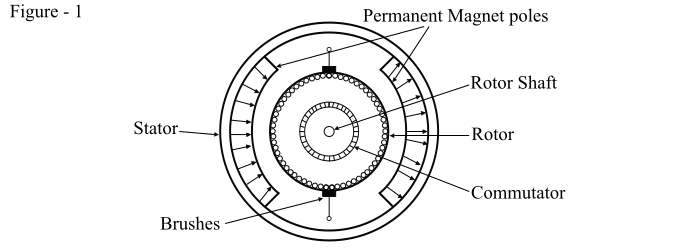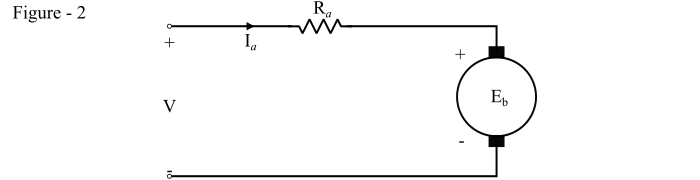# Permanent Magnet DC (PMDC) Motor ŌĆō Construction, Working, and Speed Control

A permanent magnet DC (PMDC) motor is a type of DC motor whose field poles are made up of permanent magnets. The cross-sectional view of a 2-pole permanent magnet DC Motor is shown in Figure-1In a PMDC motor, the permanent magnet poles are radially magnetised and mounted on the inner periphery of the stator of the motor. The stator also serves as a return path for the magnetic flux. The rotor of the PMDC motor has a conventional DC armature with commutator and brushes.

In a permanent magnet DC motor, the torque is produced by the interaction between axial current carrying rotor conductors and the magnetic flux produced by the permanent magnetic poles.

The equivalent circuit of a PMDC motor is shown in Figure-2. As the field flux in a permanent magnet DC motor is produced by permanent magnets, hence, the field winding is not shown in the equivalent circuit.In a conventional DC motor, the back EMF generated is given by,

$$\mathrm{­ØÉĖ_{­ØæÅ} = ­Øæś\:φ\:­Øæü … (1)}$$

And the electromagnetic torque produced is

$$\mathrm{τ_{­ØæÆ} = ­Øæś\:φ\:­ØÉ╝_{­ØæÄ} … (2)}$$

In a PMDC motor the flux is constant, thus eqn. (1) and (2) are modified as,

$$\mathrm{­ØÉĖ_{­ØæÅ} = ­Øæś_{1}\:­Øæü … (3)}$$

$$\mathrm{τ_{­ØæÆ} = ­Øæś_{1}\:­ØÉ╝_{­ØæÄ} … (4)}$$

Where, ­Øæś1 = ­Øæś φ is known as the torque constant of the PMDC motor and its value depends upon the number of field poles, armature conductors, etc.

## Speed Control of PMDC Motor

Refer the equivalent circuit of the PMDC motor, we get

$$\mathrm{­Øæē = ­ØÉĖ_{­ØæÅ} + ­ØÉ╝_{­ØæÄ}­Øæģ_{­ØæÄ} = ­Øæś_{1}\:­Øæü + ­ØÉ╝_{­ØæÄ}­Øæģ_{­ØæÄ}}$$

$$\mathrm{­Øæü =\frac{­Øæē − ­ØÉ╝_{­ØæÄ}­Øæģ_{­ØæÄ}}{­Øæś_{1}}… (5)}$$

From eq. (5), it is clear that the speed and torque of a PMDC motor can be controlled by armature voltage control method, armature rheostat control method and chopper control method.

Since the magnetic flux is constant for a PMDC motor, hence, the flux control method cannot be used for controlling the speed of a PMDC motor. Therefore, the speeds above the base speed cannot be obtained in the PMDC motors. Thus, these motors find applications where motor speeds below base speeds are required.

PMDC motors have several advantages over the conventional DC shunt motors, some of them are given below −

• PMDC motors do not require field winding, thus, they do not have field circuit copper loss.

• PMDC motors have higher efficiency as compared to conventional DC motors.

• Since field winding are not used in PMDC motors, hence, these motors are compact in sized than conventional DC motors.

• As the magnetic field is created by permanent magnets, there is no need of field excitation arrangement in case of PMDC motors.

• PMDC motors are cheaper and economical for fractional kW rated applications.

Permanent magnet DC motors suffer from the following disadvantages −

• Since the permanent magnets cannot produce high flux density, the electromagnetic torque produced per ampere in a PMDC motor is smaller.

• There is a risk of de-magnetisation of the magnetic poles which may be caused by large armature currents or excessive heating due to overloading for a long period.

• The magnetic field of a PMDC motor is present at all times even when the motor is not being used. Therefore, the motor should be totally enclosed so that no foreign magnetic material is attracted by the permanent magnets to avoid harm to the motor.

• In a PMDC motor, the armature reaction cannot be compensated, thus, the strength of the magnetic field may get weak due to the de-magnetising effect of the armature reaction.

• Since the field flux is fixed in the PMDC motor, it is difficult to control the speed of the permanent magnet DC motor.

## Applications of PMDC Motors

PMDC motors are used in several applications ranging from fractions to several hp. Some of the applications of PMDC motors are listed below −

• PMDC motors are used in automobiles to operate wind-shield wipers and washers, to raise and lower the windows, to drive the blowers for heaters & air conditioners.

• PMDC motors are used in computer drives.

• PMDC motors are used in toy industries.

• PMDC motors are used in electric tooth brushes.

• Used in portable vacuum cleaners.

• Used in food mixers.

• Also used in portable electric tools such as drills, saws, trimmers, etc.